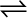7.34 The reaction, CO(g) + H2(g)CH4(g) + H2O(g)

is at equilibrium at 1300 K in a 1L flask. It also contains 0.30 mol of CO, 0.10 mol of H2 and 0.02 mol of H2O and an unknown amount of CH4 in the flask. Determine the concentration of CH4 in the mixture. The equilibrium constant, Kc for the reaction at the given temperature is 3.90.

Let the concentration of methane at equilibrium be x.

It is given that ${\mathrm{K}}_{\mathrm{c}}$= 3.90.

Therefore,

Hence, the concentration of $C{H}_{4}$ at equilibrium is